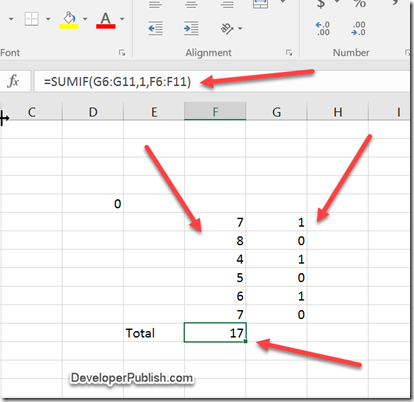How to Sum Alternate Rows/Columns cell values in Microsoft Excel ? - Developer Publish

# How to Sum Alternate Rows/Columns cell values in Microsoft Excel ?

There are times when you would have come across a scenario where you need to sum up the value of every other alternate row. You can easily do that in Microsoft Excel 2016 by using the SUMIF formula.

# How to Sum Alternate Rows/Columns cell values in Microsoft Excel 2016 ?

The SUMIF function calculates the sum of a range of data that meets the specific criteria. In our case , we are concerned with the odd row’s value.

Here’s how you do it.

Assume that the values are defined in the cell F6 to F11 and you need to find the sum of alternate or odd rows i.e F6+F8+F10.

First , add the values 1 to the next column for the cells G6 , G8 and G10 and then add the values 0 to G7 , G9 , G11.

Apply the formula

=SUMIF(G6:G11,1,F6:F11)

You should see the result in the cell where the formula is applied.